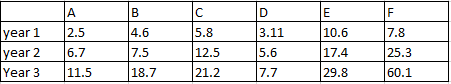### CAT 1996 Question Paper Question 2

Instructions

Direction for questions: Answer the questions based on the following information. The data given in the table shows the investment details in country ‘Fortune Land’ of companies A, B, C, D, E and F. Figures in the table are in US dollars in billions.Question 2

# What is the ratio of investments of E to F for the years 1 to 3?

Solution

Total investment of E = 57.8 Billion

Total investment of F = 93.2 Billion

Ratio = 57.8 : 93.2 = 19:31